Function Repository Resource:

# NLimit

Find the limiting value of an expression numerically

Contributed by: Wolfram Research
 ResourceFunction["NLimit"][expr,z→z0] numerically finds the limiting value of expr as z approaches z0.

## Details and Options

The expression expr must be numeric when its argument z is numeric.
ResourceFunction["NLimit"] constructs a sequence of values that approach the point z0 and uses extrapolation to find the limit.
ResourceFunction["NLimit"] is unable to recognize small numbers that should in fact be zero. Chop may be needed to eliminate these spurious residuals.
ResourceFunction["NLimit"] often fails when the limit has a power law approach to infinity.
The following options can be given:
 WorkingPrecision MachinePrecision precision to use in internal computations Direction Automatic vector giving the direction of approach "Scale" 1 initial step size in the sequence of steps "Terms" 7 number of terms used to evaluate the limit Method "EulerSum" the method used to evaluate the result "WynnDegree" 1 degree used in Wynn's epsilon algorithm
The option specifies that the approach vector to a finite limit point z0 is given by the complex number d. The default setting is equivalent to Direction-1, and computes the limit as z approaches z0 from larger values.
ResourceFunction["NLimit"] approaches infinite limit points on a ray from the origin.
The option "Scale" specifies the initial step in the constructed sequence.
For finite limit points x0, the initial step is a distance "Scale" away from x0. For infinite limit points, the initial step is a distance "Scale" away from the origin.
The accuracy of the result is generally improved by increasing the number of terms, although increased WorkingPrecision will also usually be necessary.
Possible settings for Method include:
 "EulerSum" converts sequence to a sum "SequenceLimit" constructs a sequence
The option "WynnDegree" specifies the number of iterations of Wynn's epsilon algorithm to be used by "SequenceLimit". In general, there must be at least 2(n+1) terms for n iterations.

## Examples

### Basic Examples (2)

Find the limit at zero:

 In:=Out=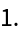Find the limit at infinity:

 In:=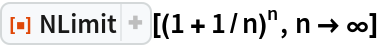Out=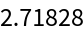### Scope (2)

The expression can be manifestly complex:

 In:=Out=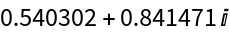The limit point can be complex:

 In:=Out=### Options (16)

#### Terms (2)

Expressions that approach their limiting value exponentially need fewer terms:

 In:=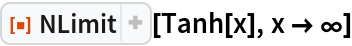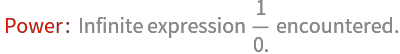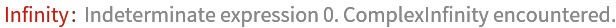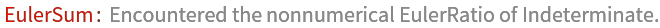Out=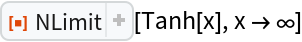In:=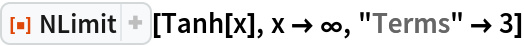Out=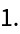Increasing the number of terms can improve accuracy:

 In:=Out=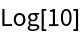Error in numerical approximation:

 In:=Out=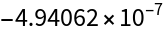Use more terms to reduce error:

 In:=Out=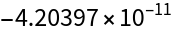#### Scale (2)

Use "Scale" to avoid regions where the expression is undefined:

 In:=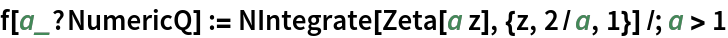In:=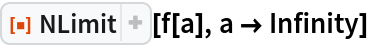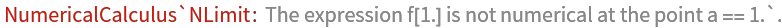Out=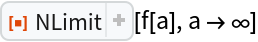The function f(x) diverges for f(1), so choose the initial step to avoid this divergence:

 In:=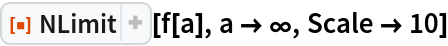Out=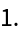#### Direction (3)

Approach 0 along the negative real axis:

 In:=Out=Approach 0 along the positive imaginary axis:

 In:=Out=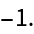Approach 0 from the 3rd quadrant, at 225°:

 In:=Out=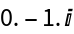#### Method (2)

An example where the default method works fairly well:

 In:=Out=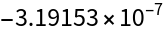Using "SequenceLimit" produces poorer results:

 In:=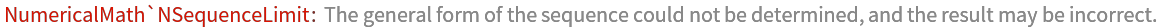Out=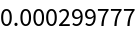An example where the default method works poorly:

 In:=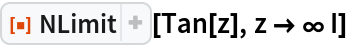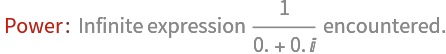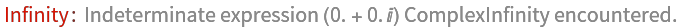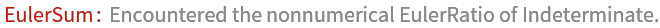Out=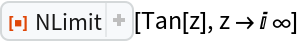Here, "SequenceLimit" produces the correct result:

 In:=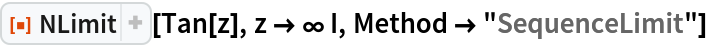Out=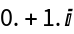#### WynnDegree (3)

When using Method"SequenceLimit", increasing "WynnDegree" may improve the accuracy of the limit:

 In:=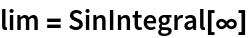Out=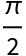Error with "WynnDegree"1:

 In:=Out=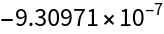Error with "WynnDegree"3:

 In:=Out=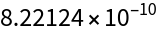#### WorkingPrecision (4)

Increasing WorkingPrecision alone does not produce a more accurate result:

 In:=Out=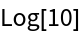Error with :

 In:=Out=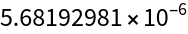Error with :

 In:=Out=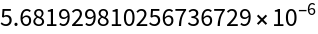To improve accuracy, the number of terms needs to be increased:

 In:=Out=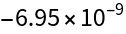### Applications (2)

Find the limit of a numerically defined function:

 In:=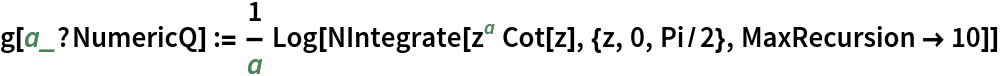In:=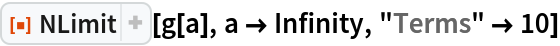Out=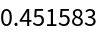Limits where parts of the expression have essential singularities:

 In:=Out=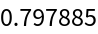In this case, the exact limit can be found:

 In:=Out=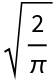Check:

 In:=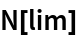Out=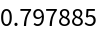### Possible Issues (1)

Limits whose value approaches infinity are sometimes unable to be computed:

 In:=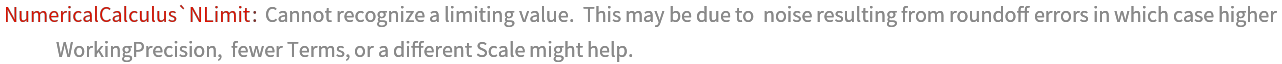Out=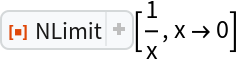## Requirements

Wolfram Language 11.3 (March 2018) or above

## Version History

• 1.0.0 – 14 March 2019